085-1263404

### My Journal

All things Mathematical
4 Oct 2018
##### Question 1

A body moving in a straight line travels distances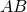,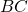,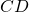of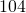ft.,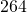ft.,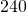ft. respectively in three successive intervals of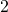secs.,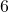secs., and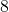secs. Show that these facts are not inconsistent with the hypothesis that the body is moving with uniform retardation. On this hypothesis that the body is moving with uniform retardation. On this hypothesis find the distance from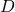to the point where the velocity vanishes and the time occupied in describing this distance.

##### Question 2

A body weighing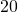lb. is placed on a rough plane inclined at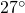to the horizontal. The angle of friction is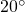. Find (a) the least force parallel to a line of greatest slope; (b) the least horizontal force, that will move the body up the plane.

##### Question 3

A uniform rod, of weight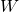lbs., is suspended in a vertical position by a string attached to it at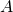. It is now drawn from the vertical by a force acting horizontally on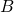, and equal to one-third the weight of the rod. Find the tension of the string and the angle it makes with vertical when the rod is in the position of equilibrium. Find also the inclination of the rod to the vertical.

##### Question 4

The parallel sides of a trapezium are of lengths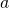and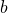respectively and are a distance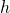apart. Find the distance of the centroid from the side.

A trapezium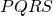is part of a rectangle. The co-ordinates of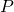,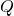,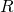,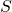are respectively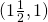;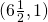;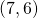;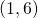and those of,.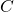,are respectively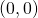;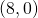;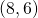;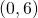. Find the centroid of the figure obtained when the trapeziumis removed from the rectangle.

##### Question 5

A placeis situated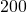miles north-west of. An aeroplane has a maximum speed ofmiles per hour in still air and a south-west wind of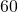m.p.h. is blowing at the level at which the plane is flying. Find the shortest time needed to fly fromtoand the compass course which the navigator must steer.

##### Question 6

An engine weighstons, of which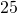tons is borne by the driving wheels. The engine is coupled to a carriage weighing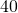tons. The resistance to the motion of the engine is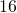lb. per ton and to the motion of the carriage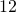lb. per ton. If the coefficient of friction between the driving wheels and the rails is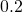, find the maximum acceleration (i.e., when the driving wheels are on the point of slipping), and the tension in the coupling between the engine and the carriage.

##### Question 7

A metal cube of sidecm. is suspended in a liquid of density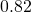grm. per c.c. so that a pair of faces are horizontal and the upper facecm. below the surface of the liquid. The atmospheric pressure is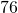cm. of mercury (density of mercury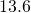grm. per c.c.). Find the resultant upward thrust on the cube due to the liquid and the total thrust on a vertical face of the cube.

##### Question 8

Two small blocks, of weightslb., andlb., are suspended from the same point by equal strings each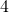ft. long. Thelb. block has a small spike in  it so that when it collides with thelb. block the two adhere together. Thelb. block is raised till the string to which it is attached is horizontal, and then let go.

Find its velocity (i) just before, (ii) immediately after it strikes thelb. block. Find in foot-pounds the decrease in kinetic energy due to the collision and the sum of the tensions in the strings immediately after the collision.

##### Question 9

Prove the formula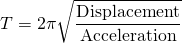for a point moving in a straight line with simple harmonic motion. If the period is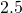sec. find the amplitude if the acceleration is not greater than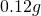.

##### Citation:

State Examinations Commission (2018). State Examination Commission. Accessed at: https://www.examinations.ie/?l=en&mc=au&sc=ru

Malone, D and Murray, H. (2016). Archive of Maths State Exams Papers. Accessed at: http://archive.maths.nuim.ie/staff/dmalone/StateExamPapers/

##### Licence:

“Contains Irish Public Sector Information licensed under a Creative Commons Attribution 4.0 International (CC BY 4.0) licence”.

The EU Directive 2003/98/EC on the re-use of public sector information, its amendment EU Directive 2013/37/EC, its transposed Irish Statutory Instruments S.I. No. 279/2005, S.I No. 103/2008, and S.I. No. 525/2015, and related Circulars issued by the Department of Finance (Circular 32/05), and Department of Public Expenditure and Reform (Circular 16/15 and Circular 12/16).

Note. Circular 12/2016: Licence for Re-Use of Public Sector Information adopts CC-BY as the standard PSI licence, and notes that the open standard licence identified in this Circular supersedes PSI General Licence No: 2005/08/01.